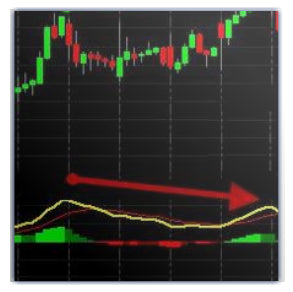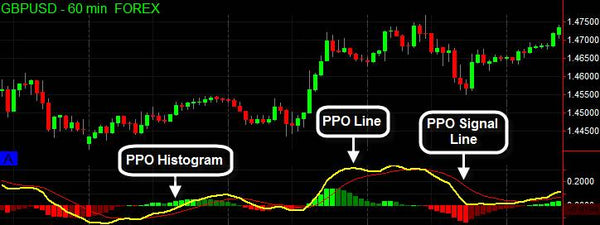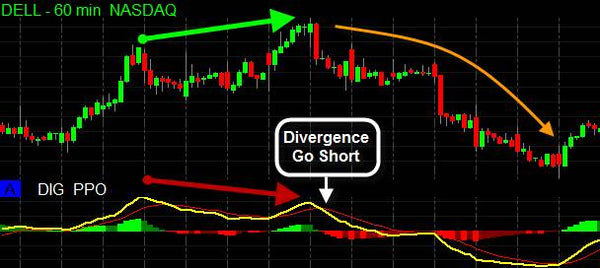# DIG Percentage Price Oscillator

• \$129.00

## What is PPO?

The percentage price oscillator – the PPO – is a momentum oscillator that measures the difference between two moving averages as a percentage of the larger moving average. The PPO is somewhat of a distant cousin to the MACD; it consists of a PPO line, a signal line, and a histogram. Entry signals are generated with crossovers of the PPO line and the signal line, the histogram, and divergences.

### How is PPO better then MACD?

The main advantage of the PPO is the way its calculations are built. The PPO is calculated as a percentage change, while the MACD measures in absolute values. This key feature makes the PPO more precise and easier to use. It also allows you to use the PPO indicator to compare stocks with different price ranges. For example, regardless of the price of a stock, a PPO result of 4 means that the short-term average is 4% above the long-term average.### GBPUSD Forex 60 Min Chart:

The yellow line represents the PPO; the thin red line is the PPO signal. The histogram represents the difference between the PPO line and the PPO signal line.
Note that for ease of use the histogram changes color according to its direction.

## How to Use the PPO?

Entry Signals: The PPO can be used to recognize a change in trend direction. When the PPO line crosses above the signal line, we have a buy long signal. This is also indicated by the histogram crossing the zero line from beneath. The same goes for short; when the PPO crosses below the signal line, the histogram crosses the zero line from above and we have a sell short signal.IBM Daily Chart: We can clearly see two nice trades; the first is a short trade when the PPO crosses below the signal. The second would have served as a stop and reverse, in this case, a very nice long buy trade when the histogram becomes positive.

Divergence: Another very common way to use the PPO indicator is divergence.
Long Divergence Example : The price action has two upper pivots, where the newer one is higher. Meanwhile, the PPO has two upper pivots where the newer one is lower. This is a powerful short signal.Dell 60 Min Chart We have a very nice short divergence, and we also have a PPO and signal cross. Together, these two signals result in a very powerful short trade.

Key Features:

• Visually enhanced.
• Histogram changes colors according to direction.